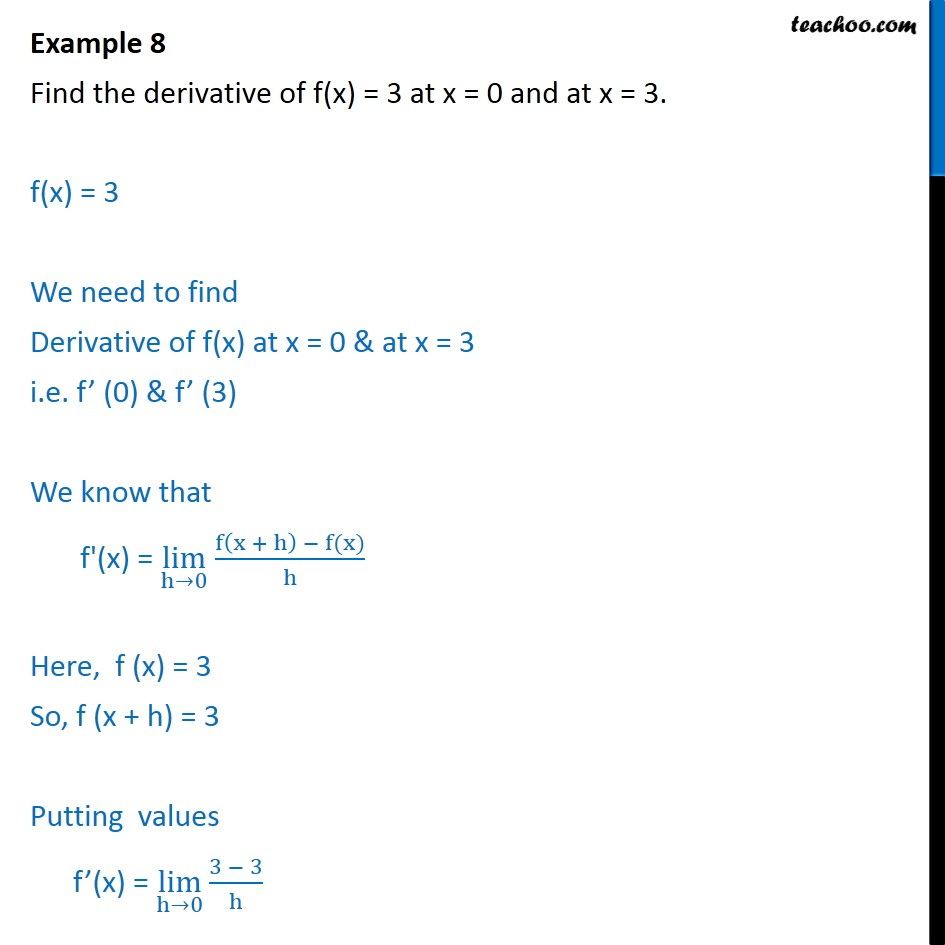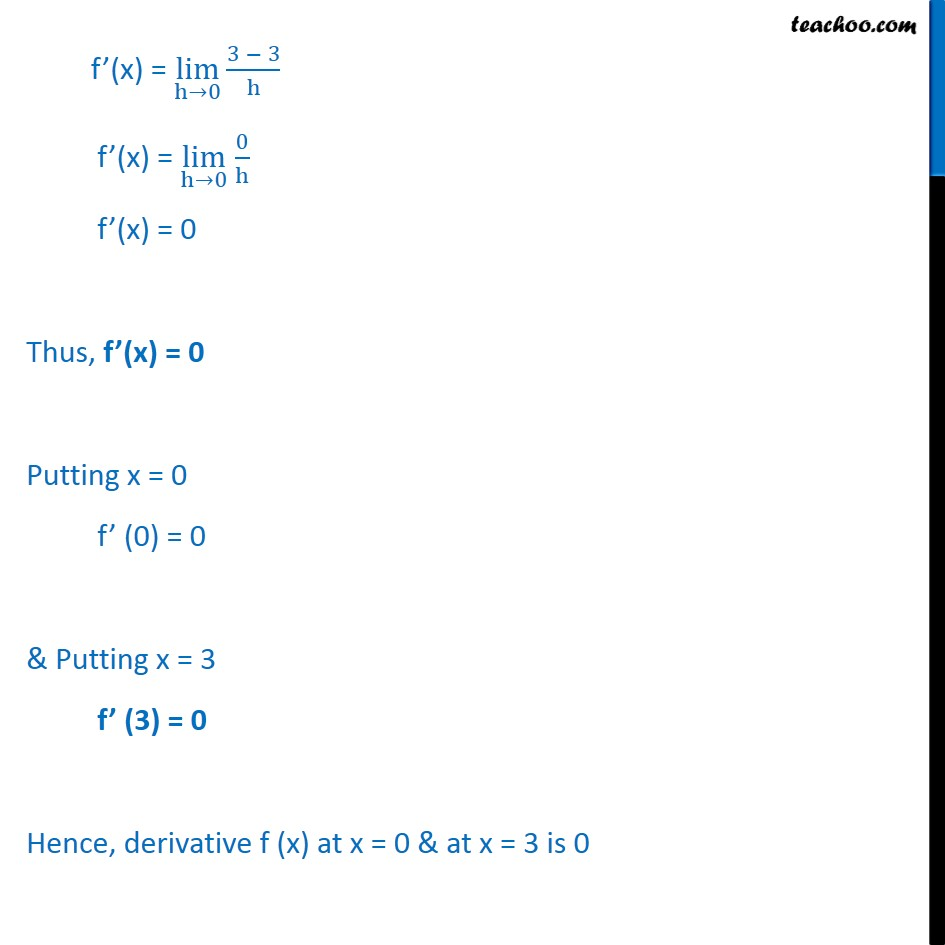Examples

Chapter 12 Class 11 Limits and Derivatives
Serial order wiseLearn in your speed, with individual attention - Teachoo Maths 1-on-1 Class

### Transcript

Example 8 Find the derivative of f(x) = 3 at x = 0 and at x = 3. f(x) = 3 We need to find Derivative of f(x) at x = 0 & at x = 3 i.e. f (0) & f (3) We know that f'(x) = lim h 0 f x + h f(x) h Here, f (x) = 3 So, f (x + h) = 3 Putting values f (x) = lim h 0 3 3 h f (x) = lim h 0 3 3 h f (x) = lim h 0 0 h f (x) = 0 Thus, f (x) = 0 Putting x = 0 f (0) = 0 & Putting x = 3 f (3) = 0 Hence, derivative f (x) at x = 0 & at x = 3 is 0